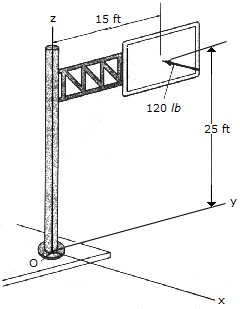# Engineering Mechanics - Force System Resultants - Discussion

### Discussion :: Force System Resultants - General Questions (Q.No.29)

29.The resultant force of a wind loading acts perpendicular to the face of the sign as shown. Replace this force by an equivalent force and couple moment acting at point O.

 [A]. F = -120i lb, M = (-3000j+1800k) lb-ft [B]. F = -120i lb, M = 3500i lb-ft [C]. F = -120i lb, M = -3500j lb-ft [D]. F = -120i lb, M = 3500k lb-ft

Explanation:

No answer description available for this question.